# Using the Monte Carlo Method to Calculate the Value of Pi

In the article about John von Neumann we mentioned that he invented the Monte Carlo method. This method is very simple in its nature. It uses the “brute force” of computing to solve a wide range of problems. It performs many (thousands of) trials to infer results such as areas or volumes of complex objects, and many other types of problems that often could not be solved otherwise. Let us show an example of how this method works – we will calculate the value of Pi.

Let’s start by drawing a quarter-circle in the unit square. Obviously, the area of the square is S = 1. The area of the quarter-circle is A = Pi*S/4. Let’s pretend that we don’t know the value of Pi. To calculate it, we will generate a large number N of random points in the unit square. By I we will denote the number of points lying inside the quarter-circle.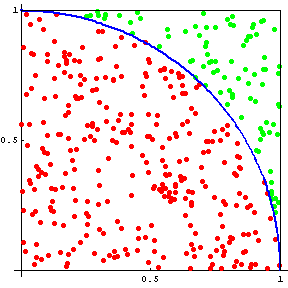As you will certainly agree, with large N the ratio I / N must be very similar to the ratio of A / S. And that’s all! From the equation I / N = A / S we can easily express that Pi = 4 * I / N. The corresponding Python program is presented below. You can easily run it using the Python app in the Creative Suite – it only has 10 lines not counting comments: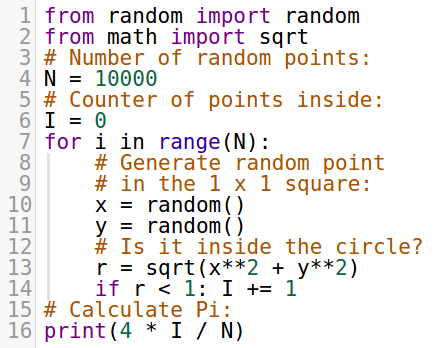Here, the function random() imported from the “random” library generates a random number between 0 and 1. The number N on line 4 defines the number of random points we want to use. We will play with it in a moment. On line 6 we define a counter for the points lying inside the quarter-circle, and this counter is increased on line 14 whenever the random point lies inside. The resulting approximate value of Pi = 4*I/N is calculated on line 16. Now let’s see some results! First, we will call the program five times with N = 1,000: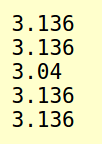These results are not very accurate, are they. So, let’s run the program five times with N = 10,000: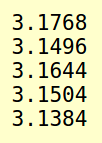Third, we will run the program five times with N = 100,000: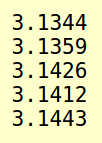And last, let’s call the program five times with N = 1,000,000: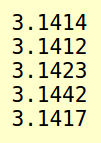We can see that the Monte Carlo method needs lots of computing power to deliver accurate results! In our case, obtaining the value of Pi accurate to two decimal digits required shooting 1,000,000 random points.